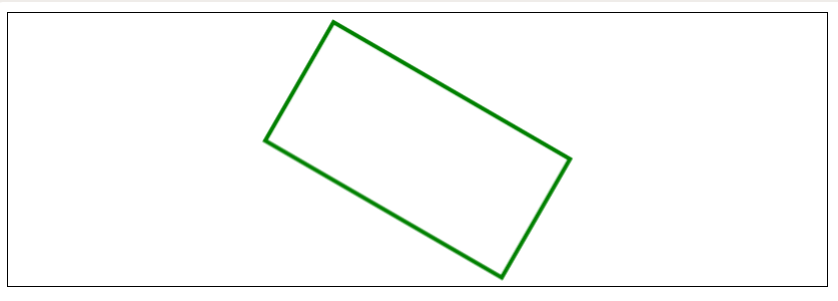Skip to content
Related Articles
Fabric.js | Rect angle Property
• Last Updated : 20 May, 2020

In this article, we are going to see how to set the angle of rotation of an object in the degree of a canvas rectangle using FabricJS. The canvas rectangle means rectangle is movable and can be stretched according to requirement. Further, the rectangle can be customized when it comes to initial stroke color, height, width, fill color, or stroke width.

Approach: To make it possible, we are going to use a JavaScript library called FabricJS. After importing the library using CDN, we will create a canvas block in the body tag that will contain our rectangle. After this, we will initialize instances of Canvas and Rectangle provided by FabricJS and use the angle property to rotate the text to the required angle and render the Canvas on the rectangle as given in the example below.

Syntax:

```fabric.Rect({
width: number,
height: number,
angle: number
}); ```

Parameters: This function accepts three parameters as mentioned above and described below:

• width: It specifies the width of rectangle.
• height: It specifies the height of rectangle.
• angle: It specifies the angle of rotation.

Example: This example uses FabricJS to create a rectangle and make it rotatable.

 ` ``<``html``> `` ` `<``head``> ``    ``<``title``> ``        ``Fabric.js | Rect angle Property``    `` ``     ` `    ````    ``<``script` `src``= ``"https://cdnjs.cloudflare.com/ajax/libs/fabric.js/3.6.2/fabric.min.js"``> ``    `` `` `` ` `<``body``> ``    ``<``canvas` `id``=``"canvas"` `width``=``"600"` `height``=``"200"``        ``style``=``"border:1px solid #000000"``> ``    `` ``     ` `    ``<``script``> ``     ` `        ``// Initiate a Canvas instance ``        ``var canvas = new fabric.Canvas("canvas"); `` ` `        ``// Initiate a Rect instance ``        ``var rectangle = new fabric.Rect({ ``            ``width: 200,``            ``height: 100,``            ``fill: '', ``            ``stroke: 'green', ``            ``strokeWidth: 3,``            ``angle: 30``        ``}); `` ` `        ``// Render the Rect in canvas ``        ``canvas.add(rectangle); ``        ``canvas.centerObject(rectangle);``    `` `` `` ` ``

Output:My Personal Notes arrow_drop_up